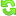Hello Learner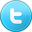Contributor
Stats
 Views : 49951 Comments : 0 Rating : 0
Like us on Facebook
Actions

# C#.NET Program to read a 4-digit integer and output the sum of its digits

By Ashok Nalam on 16 Mar 2015 | Category: C# | Tagged: number .NET
In this snippet we will write C#.NET program to read a four digit integer and output the sum of its digits. Example: If user enters number as 1234 then the output will be 1+2+3+4=9.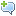Add Comment   |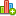Rate this resource

### Introduction

In general programming assignments people will ask to write program which asks the user for a four digit number and outputs the sum of its digits. In this snippet we tried to achieve the same using while loop.

Example:

If user enters number as 1234 then the output will be 1+2+3+4= 9.

### C# Program:

```    class SumOfDigitsProgram
{
public static void Main()
{
int sum = 0;
Console.WriteLine(" /********** Program to sum of Digits ************/");
Console.Write("Enter a number:");
int number = int.Parse(Console.ReadLine());
int tempnumber = number;
while (tempnumber > 0)
{
sum += (tempnumber % 10); // add last digit of tempnumber to sum
tempnumber /= 10; // divide tempnumber by 10 to cuts the last digit
}
Console.Write("Sum of {0} digit is {1}",number,sum);
}
}```

#### Output: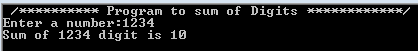Add CommentRate this resource
 Name Email WebSite